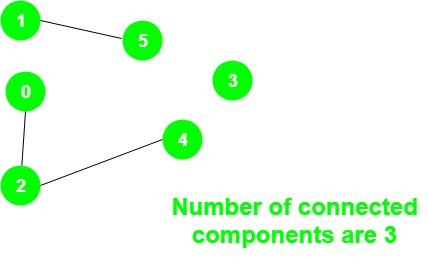# Program to count Number of connected components in an undirected graph

Given an undirected graph g, the task is to print the number of connected components in the graph.
Examples:

Input:Output:
There are three connected components:
1 – 5, 0 – 2 – 4 and 3

Approach: The idea is to use a variable count to store the number of connected components and do the following steps:

1. Initialize all vertices as unvisited.
2. For all the vertices check if a vertex has not been visited, then perform DFS on that vertex and increment the variable count by 1.

Below is the implementation of the above approach:

## CPP

 `// C++ implementation of the approach` `#include ` `using` `namespace` `std;`   `// Graph class represents a undirected graph` `// using adjacency list representation` `class` `Graph {` `    ``// No. of vertices` `    ``int` `V;`   `    ``// Pointer to an array containing adjacency lists` `    ``list<``int``>* adj;`   `    ``// A function used by DFS` `    ``void` `DFSUtil(``int` `v, ``bool` `visited[]);`   `public``:` `    ``// Constructor` `    ``Graph(``int` `V);`   `    ``void` `addEdge(``int` `v, ``int` `w);` `    ``int` `NumberOfconnectedComponents();` `};`   `// Function to return the number of` `// connected components in an undirected graph` `int` `Graph::NumberOfconnectedComponents()` `{`   `    ``// Mark all the vertices as not visited` `    ``bool``* visited = ``new` `bool``[V];`   `    ``// To store the number of connected components` `    ``int` `count = 0;` `    ``for` `(``int` `v = 0; v < V; v++)` `        ``visited[v] = ``false``;`   `    ``for` `(``int` `v = 0; v < V; v++) {` `        ``if` `(visited[v] == ``false``) {` `            ``DFSUtil(v, visited);` `            ``count += 1;` `        ``}` `    ``}`   `    ``return` `count;` `}`   `void` `Graph::DFSUtil(``int` `v, ``bool` `visited[])` `{`   `    ``// Mark the current node as visited` `    ``visited[v] = ``true``;`   `    ``// Recur for all the vertices` `    ``// adjacent to this vertex` `    ``list<``int``>::iterator i;`   `    ``for` `(i = adj[v].begin(); i != adj[v].end(); ++i)` `        ``if` `(!visited[*i])` `            ``DFSUtil(*i, visited);` `}`   `Graph::Graph(``int` `V)` `{` `    ``this``->V = V;` `    ``adj = ``new` `list<``int``>[V];` `}`   `// Add an undirected edge` `void` `Graph::addEdge(``int` `v, ``int` `w)` `{` `    ``adj[v].push_back(w);` `    ``adj[w].push_back(v);` `}`   `// Driver code` `int` `main()` `{` `    ``Graph g(5);` `    ``g.addEdge(1, 0);` `    ``g.addEdge(2, 3);` `    ``g.addEdge(3, 4);`   `    ``cout << g.NumberOfconnectedComponents();`   `    ``return` `0;` `}`

## Java

 `// Java program for above approach` `import` `java.io.*;` `import` `java.util.*;`   `public` `class` `ConnectedComponentCount` `{` `    `  `    ``private` `int` `v;` `    ``private` `int` `e;` `    ``private` `Map> adjMap;` `    ``private` `static` `Map visited;` `    `  `    ``ConnectedComponentCount(``int` `vertices)` `    ``{` `        ``v = vertices;` `        ``adjMap = ``new` `HashMap>(); ` `        ``visited = ``new` `HashMap();` `    ``}` `    `  `    ``// Function to add edges` `    ``private` `void` `addEdge(``int` `s, ``int` `d)` `    ``{` `        ``adjMap.putIfAbsent(s, ` `                     ``new` `HashSet());` `        ``adjMap.putIfAbsent(d, ` `                     ``new` `HashSet());` `        ``adjMap.get(s).add(d);` `        ``adjMap.get(s).add(s);` `        ``adjMap.get(d).add(s);` `        ``adjMap.get(d).add(d);` `        ``visited.put(s, ``0``);` `        ``visited.put(d, ``0``);` `    ``}` `    `  `    ``// To mark vertices which can be visites` `    ``private` `void` `findDFS(``int` `vertex)` `    ``{` `        `  `        ``// Mark as visited` `        ``visited.put(vertex,``1``);` `      `  `        ``// Print the vertex` `        ``System.out.println(``"vertex "` `+ vertex + ` `                                   ``" visited"``);` `        ``for``(Integer child : adjMap.get(vertex))` `        ``{` `            ``if``(visited.get(child) == ``0``){` `               ``findDFS(child);` `            ``}` `        ``}` `    ``}` `    `  `    ``// Function to print graph` `    ``private` `void` `printGraph()` `    ``{` `        ``for``(HashSet v : ` `                          ``adjMap.values())` `        ``{` `            ``System.out.println(v.toString());` `        ``}` `    ``}` `    `  `    ``// Driver Code` `    ``public` `static` `void` `main(String args[])` `    ``{` `        `  `        ``Scanner sc = ``new` `Scanner(System.in);` `        ``System.out.println("Enter the number ` `                           ``of vertices (V): ");` `        ``int` `vertices = sc.nextInt();` `        `  `        ``System.out.println("Enter the number ` `                                ``of edges (E): ");` `        ``int` `edges = sc.nextInt();` `        `  `        ``// To count total number of` `        ``// components` `        ``int` `ccCount = ``0``;` `        `  `        ``ConnectedComponentCount ccc = ` `                 ``new` `ConnectedComponentCount(` `                                      ``vertices);` `        `  `        ``// Input of edges` `        ``while` `(edges > ``0``)` `        ``{` `            ``System.out.println("Enter the ` `                                ``nodes s & d: ");` `            ``int` `s = sc.nextInt();` `            ``int` `d = sc.nextInt();` `            ``ccc.addEdge(s,d);` `            ``edges-- ;` `        ``}` `        `  `        ``// Function to print graph                      ` `        ``ccc.printGraph();` `        `  `        ``// Traversing every node ` `        ``for``(Integer vertex : visited.keySet())` `        ``{` `            `  `            ``// Check if vertex is already` `            ``// visited  or not` `            ``if``(visited.get(vertex) == ``0``)` `            ``{` `                `  `                ``// Function Call for findDFS` `                ``ccc.findDFS(vertex);` `              `  `                ``// Print Component Found` `                ``System.out.println(``"CC Found"``);` `              `  `                ``// Increase the counter` `                ``ccCount++;` `            ``}` `        ``}` `        `  `        ``// Print number of components                        ` `        ``System.out.println(``"Number of cc component: "` `                                        ``+ ccCount);` `    ``}` `}`   `//The code is contributed by Alfred Skaria`

Output

```2

```

Attention reader! Don’t stop learning now. Get hold of all the important DSA concepts with the DSA Self Paced Course at a student-friendly price and become industry ready.

My Personal Notes arrow_drop_upCheck out this Author's contributed articles.

If you like GeeksforGeeks and would like to contribute, you can also write an article using contribute.geeksforgeeks.org or mail your article to contribute@geeksforgeeks.org. See your article appearing on the GeeksforGeeks main page and help other Geeks.

Please Improve this article if you find anything incorrect by clicking on the "Improve Article" button below.

Improved By : alfiskaria